Download Presentation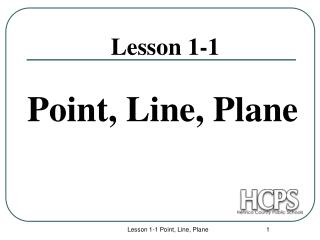Lesson 1-1

# Lesson 1-1 - PowerPoint PPT Presentation

Lesson 1-1. Point, Line, Plane. Points. Points do not have actual size. How to Sketch: Using dots How to label: Use capital letters Never name two points with the same letter (in the same sketch). . A. B. A. C. Lines.I am the owner, or an agent authorized to act on behalf of the owner, of the copyrighted work described.
Download Presentation## Lesson 1-1

An Image/Link below is provided (as is) to download presentation

Download Policy: Content on the Website is provided to you AS IS for your information and personal use and may not be sold / licensed / shared on other websites without getting consent from its author.While downloading, if for some reason you are not able to download a presentation, the publisher may have deleted the file from their server.

- - - - - - - - - - - - - - - - - - - - - - - - - - E N D - - - - - - - - - - - - - - - - - - - - - - - - - -
Presentation Transcript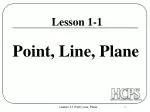### Lesson 1-1

Point, Line, Plane

Lesson 1-1 Point, Line, Plane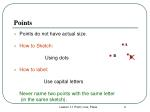Points
• Points do not have actual size.
• How to Sketch:

Using dots

• How to label:

Use capital letters

Never name two points with the same letter

(in the same sketch).

A

B

A

C

Lesson 1-1 Point, Line, Plane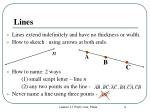Lines
• Lines extend indefinitely and have no thickness or width.
• How to sketch : using arrows at both ends.
• How to name: 2 ways

(1) small script letter – line n

(2) any two points on the line -

• Never name a line using three points -

n

A

B

C

Lesson 1-1 Point, Line, Plane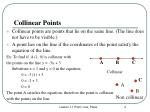Collinear Points
• Collinear points are points that lie on the same line. (The line does not have to be visible.)
• A point lies on the line if the coordinates of the point satisfy the equation of the line.

Ex: To find if A (1, 0) is collinear with

the points on the line y = -3x + 3.

Substitute x = 1 and y = 0 in the equation.

0 = -3 (1) + 3

0 = -3 + 3

0 = 0

The point A satisfies the equation, therefore the point is collinear

with the points on the line.

A

B

C

Collinear

C

A

B

Non collinear

Lesson 1-1 Point, Line, Plane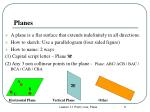Planes
• A plane is a flat surface that extends indefinitely in all directions.
• How to sketch: Use a parallelogram (four sided figure)
• How to name: 2 ways

(1) Capital script letter – Plane M

(2) Any 3 non collinear points in the plane - Plane: ABC/ ACB / BAC / BCA / CAB / CBA

A

M

B

C

Horizontal Plane

Vertical Plane

Other

Lesson 1-1 Point, Line, Plane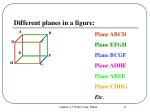Different planes in a figure:

A

B

Plane ABCD

Plane EFGH

Plane BCGF

Plane ABFE

Plane CDHG

Etc.

D

C

E

F

H

G

Lesson 1-1 Point, Line, Plane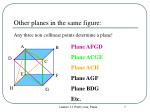Other planes in the same figure:

Any three non collinear points determine a plane!

Plane AFGD

Plane ACGE

Plane ACH

Plane AGF

Plane BDG

Etc.

Lesson 1-1 Point, Line, Plane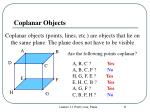Coplanar Objects

Coplanar objects (points, lines, etc.) are objects that lie on the same plane. The plane does not have to be visible.

Are the following points coplanar?

A, B, C ?

Yes

A, B, C, F ?

No

H, G, F, E ?

Yes

E, H, C, B ?

Yes

A, G, F ?

Yes

C, B, F, H ?

No

Lesson 1-1 Point, Line, Plane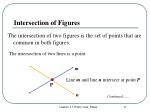Intersection of Figures

The intersection of two figures is the set of points that are common in both figures.

The intersection of two lines is a point.

m

Line m and line n intersect at point P.

P

n

Continued…….

Lesson 1-1 Point, Line, Plane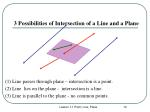3 Possibilities of Intersection of a Line and a Plane

(1) Line passes through plane – intersection is a point.

(2) Line lies on the plane - intersection is a line.

(3) Line is parallel to the plane - no common points.

Lesson 1-1 Point, Line, PlaneIntersection of Two Planes is a Line.

B

P

A

R

Plane P and Plane R intersect at the line

Lesson 1-1 Point, Line, Plane